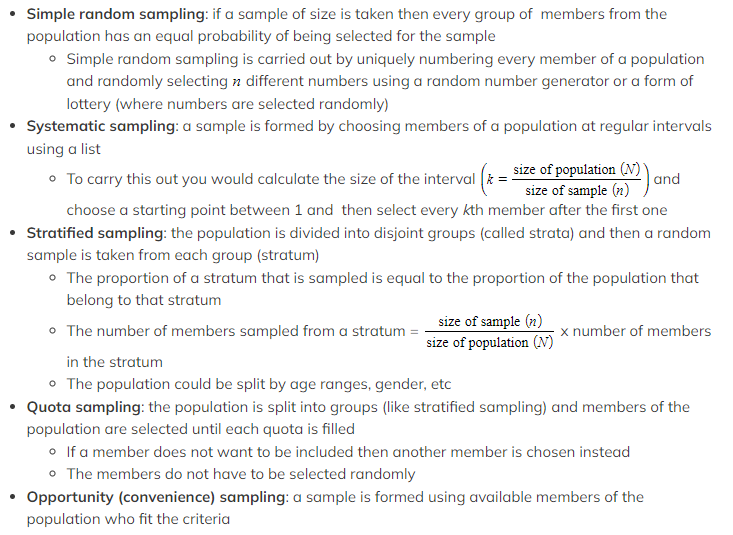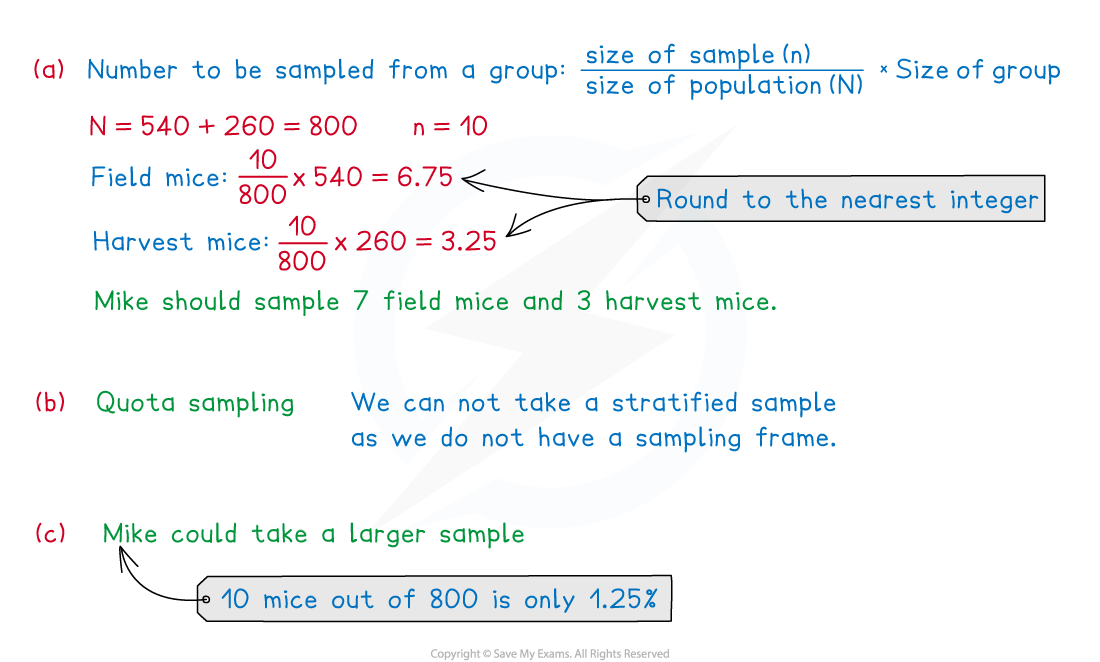# AQA A Level Maths: Statistics复习笔记1.1.1 Sampling & Data Collection

### Types of Data

#### What are the different types of data?

• Qualitative data is data that is usually given in words not numbers to describe something
• For example: the colour of a teacher's car
• Quantitative data is data that is given using numbers which counts or measures something
• For example: the number of pets that a student has
• Discrete data is quantitative data that needs to be counted
• Discrete data can only take specific values from a set of (usually finite) values
• For example: the number of times a coin is flipped until a tails is obtained
• Continuous data is quantitative data that needs to be measured
• Continuous data can take any value within a range of infinite values
• For example: the height of a student
• Age can be discrete or continuous depending on the context or how it is defined
• If you mean how many years old a person is then this is discrete
• If you mean how long a person has been alive then this is continuous

#### What other key words do I need to know?

• The population refers to the whole set of things which you are interested in
• For example: if a vet wanted to know how long a typical French bulldog slept for in a day then the population would be all the French bulldogs in the world
• A sample refers to a subset of the population which is used to collect data from
• For example: the vet might take a sample of French bulldogs from different cities and record how long they sleep in a day
• A sampling frame is a list of all members of the population
• For example: a list of employees’ names within a company
• A population parameter is a numerical value which describes a characteristic of the population
• These are usually unknown
• For example: the mean height of all 16-year-olds in the UK
• A sample statistic is a value computed using data from the sample
• These are used to estimate population parameters
• For example: the mean height of 200 16-year-olds from randomly selected cities in the UK

### Sampling Techniques

#### What are the differences between a census and sampling?

• A census collects data about all the members of a population
• For example: the Government in England does a national census every 10 years to collect data about every person living in England at the time
• The main advantage of a census is that it gives fully accurate results
• The disadvantages of a census are:
• It is time consuming and expensive to carry out
• It can destroy or use up all the members of a population when they are consumables (imagine a company testing every single firework)
• Sampling is used to collect data from a subset of the population
• The advantages of sampling are:
• It is quicker and cheaper than a census
• It leads to less data needing to be analysed
• The disadvantages of sampling are:
• It might not represent the population accurately
• It could introduce bias

### What sampling techniques do I need to know?Sampling Critique

#### When should each sampling technique be used or avoided?

• Simple random sampling: this should be used when you want a random sample to avoid bias
• Useful when you have a small population or want a small sample (such as children in a class)
• This can not be used if it is not possible to number or list all the members of the population (such as fish in a lake)
• Systematic sampling: this should be used when you want a random sample from a large population
• Useful when there is a natural order (such as a list of names or a conveyor belt of items)
• In order for the sample to be random the sampling frame needs to be random
• This can not be used if it is not possible to number or list all the members of the population (such as penguins in Antarctica)
• Stratified sampling: this should be used when the population can be split into obvious groups of members (where members within a group have a common characteristic)
• Useful when there are very different groups of members within a population
• The sample will be representative of the population structure
• The members selected from each stratum are chosen randomly
• This can not be used if the population can not be split into groups or if the groups overlap
• Quota sampling: this should be used when a small sample is needed to be representative of the population structure
• Useful when collecting data by asking people who walk past you in a public place or when a sampling frame is not available
• This can introduce bias as some members of the population might choose not to be included in the sample
• Opportunity (convenience) sampling: this should be used when a sample is needed quickly
• Useful when a list of the population is not possible
• This is unlikely to be representative of the population structure

#### What are the main criticisms of sampling techniques?

• Most sampling techniques can be improved by taking a larger sample
• Sampling can introduce bias - so you want to minimise the bias within a sample
• To minimise bias the sample should be random
• A sample only gives information about those members
• Different samples may lead to different conclusions about the population

#### Worked Example

Mike is a biologist studying mice in an open enclosure. He has access to approximately 540 field mice and 260 harvest mice. Mike wants to sample 10 mice and he wants the proportions of the two types of mice in his sample to reflect their respective proportions of the population.

(a)Calculate the number of field mice and harvest mice that Mike should include in his sample.
(b)Given that Mike does not have a list of all mice in the enclosure, state the name of this sampling method.
(c)Suggest one way in which Mike could improve his sampling method.#### Exam Tip

• Use common sense when answering questions on this topic. The best way to get a deeper understanding of sampling is to read real articles in the news and think about the sampling methods that have been used.
• Stratified and quota sampling seem similar, but the main difference is stratified involves randomly selecting the members within each stratum.